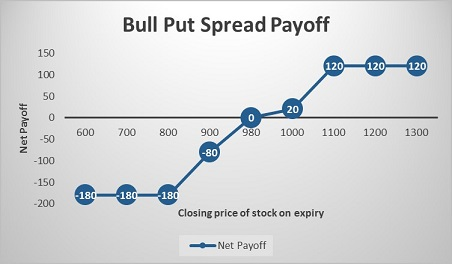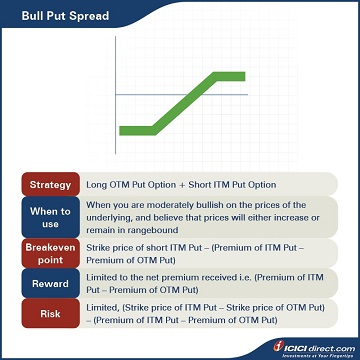It is important to understand here that Put writing brings with it the risk of sizeable losses for the trader if the value of the stock falls to a level substantially lower than the strike price. As the trader is left with no option but to buy the stock at the Put strike price, he ends up in the red.

However, this risk is lessened in the case of a Bull Put Spread as here, the trader simultaneously purchases a Put at a lower price. This lowers the premium received, but also the risk involved. Read on to know more.

## Looking at the Bull Put Spread

In the Bull Put Spread strategy, the investor buys an OTM (Out-of-the-Money) Put Option (of a lower strike price) and simultaneously sells an ITM (In-the-Money) Put Option (of a higher strike price), with the same expiry, on the same underlying asset and involving the same number of Options. This strategy is similar to a Bull Call Spread but is adopted so as to receive a net cash inflow at the start of trade, as the premium paid is less than that which is received.

Abhinav’s manager tells him to use this strategy if he is moderately bullish about prices of the underlying and believes that prices will either increase or remain rangebound. With this position, he can protect the downside risk of the Put sold (obligation to buy the asset) by purchasing a Put of a higher strike price (or the right to sell the asset).

 Did you know?  Bull Put Spread is known as credit spread due to net cash inflow.
• Maximum loss will be incurred when the spot price falls below the lower strike price, i.e., when Abhinav can exercise the Option.
• Maximum profit will be incurred when Options are not exercised, and the spot price is higher than the strike price of the short Put. In this case, Abhinav will receive the net premium amount.

Strategy: Long OTM Put Option (Leg 1) + Short ITM Put Option (Leg 2)

When to use: This should be used when you are moderately bullish on the prices of the underlying and believe that prices will either increase or remain rangebound

Breakeven: Strike price of short ITM Put – (Premium of ITM Put – Premium of OTM Put)

Maximum risk: Limited (Strike price of ITM Put – Strike price of OTM Put) – (Premium of ITM Put – Premium of OTM Put)

Let’s understand the Bull Put Spread strategy with an example:

Assume that the spot price of ABC Ltd. is Rs. 1,000. Abhinav sells an ABC Ltd. ITM Put of strike price Rs. 1,100 at Rs. 140, and buys an OTM Put Option of strike price Rs. 800 at Rs. 20. He receives a total premium of Rs. 140 – Rs. 20 = Rs. 120, and this will be the maximum profit he can make. He will start losing money if the stock moves beyond the lower strike price, i.e., Rs. 800.

Let’s look at the cash flow in various scenarios here:

 Closing price of stock on expiry (Rs.) Payoff from ITM Put Option (A) (Rs.) Payoff from OTM Put Option (B) (Rs.) Net payoff (A+B)        (Rs.) 600 – 360 180 – 180 700 – 260 80 – 180 800 – 160 – 20 – 180 900 – 60 – 20 – 80 980 20 – 20 0 1000 40 – 20 20 1100 140 – 20 120 1200 140 – 20 120 1300 140 – 20 120

Examining the payoff will give you a fair idea of how we have arrived at the above values.

### If the stock closes at Rs. 800 on expiry: Leg 1 expires OTM while leg 2 expires ITM

Leg 1: The premium paid on the OTM Put Option of strike price Rs. 800 = Rs. 20

Premium received on OTM Put Option of strike price Rs. 800 at expiry = Max {0, (Strike price – Spot price)} = Max {0, (800 – 800)} = Max (0,0) = 0

So, the payoff from the OTM Put Option = Premium received – Premium paid = 0 – 20 = – Rs. 20

Leg 2: The premium received on the ITM Put Option of strike price Rs. 1100 = Rs. 140

The premium paid on ITM Put Option of strike price Rs. 1100 at expiry = Max {0, (Strike price – Spot price)} = Max {0, (1100 – 800)} = Max (0, 300) = Rs. 300

So, the payoff from the ITM Put Option = Premium received – Premium paid =140 – 300 = – Rs. 160

Net payoff = Payoff from ITM Put Option + Payoff from OTM Put Option = (– 160) + (– 20) = – Rs. 180

### If the stock closes at Rs. 980 on expiry: Leg 1 expires OTM while Leg 2 expires ITM

Leg 1: The premium paid on the OTM Put Option of strike price Rs. 800 = Rs. 20

The premium received on OTM Put Option of strike price Rs. 800 at expiry = Max {0, (Strike price – Spot price)} = Max {0, (800 – 980)} = Max (0,0) = 0

So, payoff from the OTM Put Option = Premium received – Premium paid = 0 – 20 = – Rs. 20

Leg 2: Premium received on the ITM Put Option of strike price Rs. 1100 = Rs. 140

Premium paid on ITM Put Option of strike price Rs. 1100 at expiry = Max {0, (Strike price – Spot price)} = Max {0, (1100 – 980)} = Max (0, 120) = Rs. 120

So, payoff from the ITM Put Option = Premium received – Premium paid = 140 – 120 = Rs. 20

Net payoff = Payoff from ITM Put Option + Payoff from OTM Put Option = 20 + (– 20) = 0

### If the stock closes at 1200 on expiry: Both legs expire OTM

Leg 1: Premium paid on the OTM Put Option of strike price Rs. 800 = Rs. 20

Premium received on OTM Put Option of strike price Rs. 800 at expiry = Max {0, (Strike price – Spot price)} = Max {0, (800 – 1200)} = Max (0,0) = 0

So, payoff from the OTM Put Option = Premium received – Premium paid = 0 – 20 = – Rs. 20

Leg 2: Premium received on the ITM Put Option of strike price Rs. 1100 = Rs. 140

Premium paid on ITM Put Option of strike price Rs. 1100 at expiry = Max {0, (Strike price – Spot price)} = Max {0, (1100 – 1200)} = Max (0, – 100) = 0

So, payoff from the ITM Put Option = Premium received – Premium paid = 140 – 0 = 140

Net payoff = Payoff from ITM Put Option + Payoff from OTM Put Option = 140 + (– 20) = Rs.120## Summary• A Bull Put Spread strategy involves an investor buying an OTM Put Option (lower strike price) and simultaneously selling an ITM Put Option (higher strike price), with the same expiry, on the same underlying asset and involving the same number of Options.
• This strategy is used when the trader is moderately bullish on the prices of the underlying, and believes that the prices will either increase or remain in a particular range.
• Breakeven: Strike price of short ITM Put – (Premium of ITM Put – Premium of OTM Put)